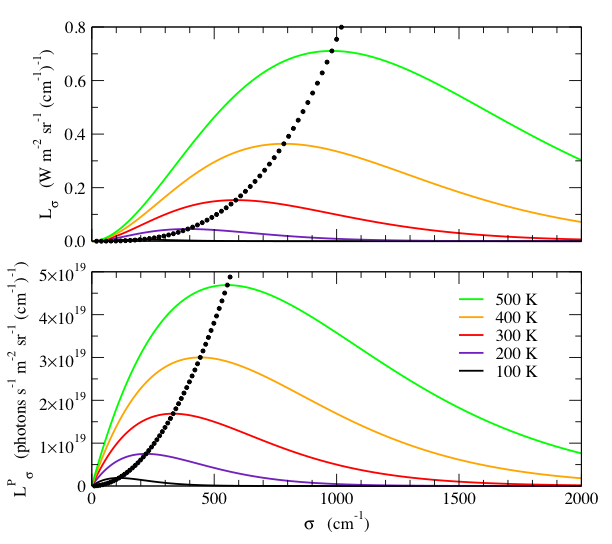Blackbody Calculator

Units of Wavenumbers

(click on equations to view enlarged)

Yet a third spectral unit, commonly used in spectroscopy, is wavenumber, the number of waves per cm: σ = ν /100c  cm-1.  Converting (1) to these units gives.(13)Again, the peak is where the derivative with respect to wavenumber vanishes:so.                                            (14)The peak value is.                    (15)The spectral photon radiance is found by dividing Lσ by the energy of a photon, 100hcσ :.      (16)We next find the wavenumber at the peak of the spectral photon radiance:and.                                            (17)The peak spectral photon radiance is.                    (18)Fig. 3 shows plots of Lσ and LσP for various temperatures.  Note again the important difference between the spectral radiance and spectral photon radiance.Fig. 3- Spectral radiance, Lσ , (top) and the spectral photon radiance, LσP, (bottom) as a function of wavenumber, σ, for various temperatures.  The small black dots indicate the wavenumber and value of the peak, at 10 K temperature intervals.  Note that Lσ and  LσP have different wavenumber dependences.  Although the peak wavenumber is proportional to T for both quantities, Lσ peaks at a higher wavenumber than LσP.  Furthermore, the peak value of Lσ increases as 3, whereas the peak value of LσP increases as 2.Calculation of a Blackbody Radiance Units of Frequency Units of Wavelength Units of Wavenumbers Radiance: Integrating the Planck Equation In-band Radiance: Integrating the Planck Equation over a Finite Range Appendix A: Algorithms for Computing In-band Radiance Appendix B: The Doppler Effect Appendix C: Summary of Formulas References Blackbody Calculator Print Version